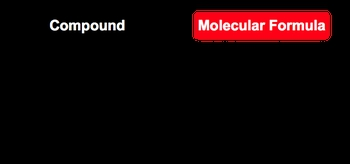Start typing, then use the up and down arrows to select an option from the list.## GOB Chemistry

Learn the toughest concepts covered in your GOB - General, Organic, and Biological Chemistry class with step-by-step video tutorials and practice problems.

Chemical Reactions & Quantities

# Molecular Formula

The molecular formula gives the actual number of atoms.

Molecular Formula
1
concept

## Molecular Formula2m
Play a video:
recall that the molecular formula gives the actual number of different elements in a given compound, meaning it gives us the rial formula for the compound. The empirical formula, on the other hand, just gives us the relative number of those different elements in that same compound. Up to this point, we've learned to calculate the empirical formula when given either the masses or the percentages of those elements within that compound. But how exactly would we determine the molecular formula for those same compounds? Well, here we have a chart, and in his church we're talking about three compounds glucose octane and salicylic acid. We're going to say that they are empirical formulas are giving us ch 20 remember that there are sub scripts of one and one there we have C four, H nine and C 78603 In order to determine their molecular formulas, we can use end factors, and factors are just numbers that we can multiply the empirical formula by in order to determine the molecular formula. So, for example, glucose has an end factor of six. That means I will multiply each of the sub scripts of the empirical formula by six, and that will give me my molecular formula so that be six carbons, 12 hydrogen and six oxygen's. So the molecular formula glucose is C 68 12 Obtain. It has an n factor off to so multiplying those sub scripts gives me see eight h 18 and then finally, salicylic acid has a factor of one, which means that the molecular formula empirical formula are the same. So just remember, when you're given the end factor, just multiply the empirical formula by it and you'll determine what your molecular formula will be. But what happens when we don't have the end factor? How do we determine it in the next series of videos will go through the different steps required to find the different end factors for any given compound once you've determined its empirical formula.2
example

## Molecular Formula Example 15m
Play a video:
3
Problem

What is the molecular formula for the following compound?

Empirical Formula: NPCl                    Molar Mass:347.64 g/mol

4
Problem

Cortisol (MW = 362.47 g/mol), a known steroid hormone, is found to contain 69.6% carbon, 8.34% hydrogen, and 22.1% oxygen by mass. What is its molecular formula?

5
Problem

Elemental analysis of a pure compound indicated that the compound had 72.2% C, 8.50% H and the remainder as O. If 0.250 moles of the compound weighs 41.55 g, what is the molecular formula of the compound?